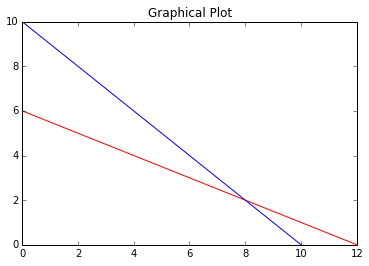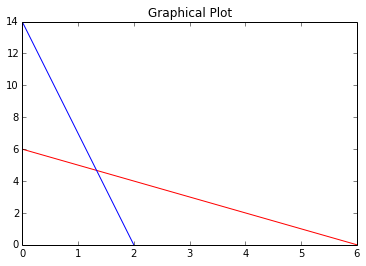# Linear Progrmming¶

## Example 16.1 Page 200¶

In :
#initiation of variable
#result
print"The data of the problem are summarized below : ";
print"Machine                   Products           Limit on ";
print"                        P1         P2       machine hours";
print"Lathe                    5         10            60";
print"Milling                  4          4            40";
print"Profit/unit              6          8";
print"Let X1 be the production volume of the product.P1, and";
print"X2 be the production volume of the product,P2.";
print"The corresponding linear programming model to determine the production volume of each product such that the total profit is maximized is as shown below : ";
print"maximize Z = 6*X1 + 8*X2";
print"subject to";
print"5*X1+10*X2 <= 60"
print"4*X1+4*X2 <= 40"
print"X1,X2 >= 0"

The data of the problem are summarized below :
Machine                   Products           Limit on
P1         P2       machine hours
Lathe                    5         10            60
Milling                  4          4            40
Profit/unit              6          8
Let X1 be the production volume of the product.P1, and
X2 be the production volume of the product,P2.
The corresponding linear programming model to determine the production volume of each product such that the total profit is maximized is as shown below :
maximize Z = 6*X1 + 8*X2
subject to
5*X1+10*X2 <= 60
4*X1+4*X2 <= 40
X1,X2 >= 0


## Example 16.2 Page 200¶

In :
#initiation of variable
print"Let X1 be the No. of packets of food type1 suggested for babies, and";
print"X2 be the No. of packets of food type1 suggested for babies.";
print"The corresponding linear programming model to determine the No. of packets of each food type to be suggested for babies with the minimum cost such that the minimum daily required vitamin in each food type is satisfied is as shown below : ";
print"maximize Z = 2*X1 + 3*X2";
print"subject to";
print"X1+X2 >= 6"
print"7*X1+X2 >= 14";
print"X1,X2 >= 0";

Let X1 be the No. of packets of food type1 suggested for babies, and
X2 be the No. of packets of food type1 suggested for babies.
The corresponding linear programming model to determine the No. of packets of each food type to be suggested for babies with the minimum cost such that the minimum daily required vitamin in each food type is satisfied is as shown below :
maximize Z = 2*X1 + 3*X2
subject to
X1+X2 >= 6
7*X1+X2 >= 14
X1,X2 >= 0


## Example 16.3 Page201¶

In :
#initiation of variable
%pylab inline
import matplotlib.pyplot as plt
print"Given the following LP model :"
print"maximize Z = 6*X1 + 8*X2";
print"subject to";
print"5*X1+10*X2 <= 60";
print"4*X1+4*X2 <= 40";
print"X1,X2 >= 0";
print"The introduction of non-negative constraints X1>=0 and X2>=0 will eliminate the 2nd, 3rd and 4th quadrants of XY plane.";
print"Compute the cordinates to plot equations relting to the constraints on the XY plane as shown below : ";
print"5*X1+10*X2 <= 60";
print"When X1=0 : X2=6";
print"When X2=0 : X1=12";
plt.plot([0,12],[6,0],'r')
plt.plot([10,0],[0,10])
plt.title('Graphical Plot')
plt.show()
print "Consider the 2nd constraint in the form : in blue ";
print "4*X1+4*X2 <= 40";
print "When X1=0 : X2=10";
print "When X2=0 : X1=10";
print "The closed polygon is the feasible region at each of the corner points of the closed polygon is computed as follows by substituting its coordinates in the objective function :";
ZA=6*0+8*0;
ZB=6*10+8*0;
ZC=6*8+8*2;
ZD=6*0+8*6;
print "Since the type of the objective function is maximization, the solution corresponding to the maximum Z value should be selected as the optimum solution. The Z value is maximum for the corner point C. Hence, the corresponding solution is ";
print "X1 = 8  X2 = 2 and Z(Optimum) is",ZC;

Populating the interactive namespace from numpy and matplotlib
Given the following LP model :
maximize Z = 6*X1 + 8*X2
subject to
5*X1+10*X2 <= 60
4*X1+4*X2 <= 40
X1,X2 >= 0
The introduction of non-negative constraints X1>=0 and X2>=0 will eliminate the 2nd, 3rd and 4th quadrants of XY plane.
Compute the cordinates to plot equations relting to the constraints on the XY plane as shown below :
5*X1+10*X2 <= 60
When X1=0 : X2=6
When X2=0 : X1=12Consider the 2nd constraint in the form : in blue
4*X1+4*X2 <= 40
When X1=0 : X2=10
When X2=0 : X1=10
The closed polygon is the feasible region at each of the corner points of the closed polygon is computed as follows by substituting its coordinates in the objective function :
Since the type of the objective function is maximization, the solution corresponding to the maximum Z value should be selected as the optimum solution. The Z value is maximum for the corner point C. Hence, the corresponding solution is
X1 = 8  X2 = 2 and Z(Optimum) is 64


## Example 16.4 Page 203¶

In :
#initiation of variable
%pylab inline
import matplotlib.pyplot as plt
print"Given the following LP model :"
print "minimize Z = 2*X1 + 3*X2";
print"subject to";
print"X1+X2 >= 6";
print"7*X1+X2 >= 14";
print"X1,X2 >= 0";
print"The introduction of non-negative constraints X1>=0 and X2>=0 will eliminate the 2nd, 3rd and 4th quadrants of XY plane.";
print"Compute the cordinates to plot equations relting to the constraints on the XY plane as shown below : ";
print"X1+X2 = 6";
print"When X1=0 : X2=6";
print"When X2=0 : X1=6";
plt.plot([0,6],[6,0],'r')
plt.plot([2,0],[0,14])
plt.title('Graphical Plot')
plt.show()
print"Consider the 2nd constraint in the form  (in blue):";
print"7*X1+X2 = 14";
print"When X1=0 : X2=14";
print"When X2=0 : X1=2";
print"The Optimum solution will be in any one of the corners A, B and C";
print"The objective function value at each of these corner points of the feasible solution space is computed as fllows by substituting its coordinates in the objective function."
ZA=2*0+3*14;
ZB=2*(4.0/3)+3*(14.0/3);
ZC=2*6+3*0;

#result
print"Since the type of the objective function is minimization, the solution corresponding to the minimum Z value should be selected as the optimum solution. The Z value is minimum for the corner point C. Hence, the corresponding solution is ";
print "X1 = 6  X2 = 0 and Z(Optimum) =",ZC

Populating the interactive namespace from numpy and matplotlib
Given the following LP model :
minimize Z = 2*X1 + 3*X2
subject to
X1+X2 >= 6
7*X1+X2 >= 14
X1,X2 >= 0
The introduction of non-negative constraints X1>=0 and X2>=0 will eliminate the 2nd, 3rd and 4th quadrants of XY plane.
Compute the cordinates to plot equations relting to the constraints on the XY plane as shown below :
X1+X2 = 6
When X1=0 : X2=6
When X2=0 : X1=6Consider the 2nd constraint in the form  (in blue):
7*X1+X2 = 14
When X1=0 : X2=14
When X2=0 : X1=2
The Optimum solution will be in any one of the corners A, B and C
The objective function value at each of these corner points of the feasible solution space is computed as fllows by substituting its coordinates in the objective function.
Since the type of the objective function is minimization, the solution corresponding to the minimum Z value should be selected as the optimum solution. The Z value is minimum for the corner point C. Hence, the corresponding solution is
X1 = 6  X2 = 0 and Z(Optimum) = 12

In [ ]:
Example 16.5 Page